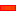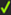﻿ Modelling | Add Math FB

# Modelling | Add Math FB

### Why use a Maths FB?

A Math FB allows you to develop new functions that may not be available with other FBs.Modelling FB toolbar > Maths FB [Toolbar is Above Graphic-Area]:

Dialog-Box:

Note: The Calculator tool may be behind the Math FB dialog-box

STEP 1: Add the Maths FB to the graphic-area

 1 Click the Add Maths FB in the Kinematics FB Toolbar.
 2 Click in the graphic-area

The Maths FB is now in the graphic-area.

How to edit:

STEP 2: Open the Maths FB dialog-box:

 1 Double-click a Maths FB in the graphic-area

or

 1

####Input-Connectors and Output-ConnectorsInput-Connectors Click the Add Input buttonto add an input-connectorAdd as many input-connectors as there are parameters and variables in the equation.Output-Connectors Click the Add Output buttonto add an output-connectorAdd as many output-connectors as there are functions / equations you need to output. For each output-connector, you can write an equation for three Data Channels(top image). How many input and output-connectors? By example, to calculate Power from Torque × Velocity P =  Ʈ . ω Power is the 'output'; Torque and Velocity are the 'inputs'. Thus, you need two input-connectors and one output-connector.

####Data from Input-Connectors, Data at Output-Connectors, Units, and Valid EquationsWire Number  and Data-Channels

Together we identify each wire and and each data channel in an equation as:

'Data-Channel(Wire-Number)'

Wire-Number

Each wire [input-connector] has a number; its 'wire-number', which start at 0

 • 0 = input-connector / wire-number 1
 • 1 = input-connector / wire-number 2
 • 2 = input-connector / wire-number 3

Data-Channel

Each wire has 3[three] data-channels.

Each data-channel has a letter - p, v, and a [even if the data at the input is not a motion-value.

 • p = Data-Channel 1
 • v = Data-Channel 2
 • a = Data-Channel 3

Each variable in an equation is identified as

Data-Channel(Wire-Number)

Example2

p(0) : p = Data-channel 1, (0)  = input-connector 1

a(2) : a = Data-channel 3,  (2) = input-connector 3

v(1) : v = Data-channel 2,  (1) =  input-connector 2Equations for each Output-Connectors

There is one output-connector when you add the Maths FB to the graphic-area. Each output-connector has  three data-channels.

In the image, we can see that we can write up to six equations [for two output-connectors].

Three equations for each of the two output-connectors [0,1]The top Output-Connector u is '0'. We can write three equations, one to each 'Output : Data-Channel # 0, 1 & 2

The next Output-Connector u is '1'. We can write three equations, one to each 'Output : Data-Channel # 3, 4 & 5.Output Data-Types drop-down box.

All output-connectors must have the same data-type.

 1 Select the 'Output Data-Type' fin the drop-down box.
 2 Click the 'Update' button at the bottom of the dialog.

For example, if you select 'Linear Coordinates', the

 • Data Output on Data-Channels 0, 3, 6, 9,... is 'Dis', with units of 'm'
 • Data Output on Data-Channels 1, 4, 7, 10,.. is 'Vel', with units of 'm/s'
 • Data Output on Data-Channels 2, 5, 8, 11,...' is 'Acc', with units of 'm/s/s'

Notes:

The Units inside the Maths FB are SI units: kilogram, metres, seconds, ...

Valid Equations

Click the 'Update' after you edit the equation to find if the syntax is correct or not correct.

The syntax is corrected in some way if it is not correct.F or example, an equation may not have the correct number of parentheses. The reason is given as a message in the Feedback-Area.

Theat the left of each equation shows whether the syntax of the equation is correct.

Each output-connector has one equation for each Data-Channel.

For example: the equation : v(0)*p(1).

It multiplies:

 • channel #2 on input-connector wire #1 ... v(0)

by

 • channel #1 on input-connector wire #2 ... p(1)

Tutorial and Reference Help Files for MechDesigner and MotionDesigner 13.2 + © Machine, Mechanism, Motion and Cam Design Software by PSMotion Ltd